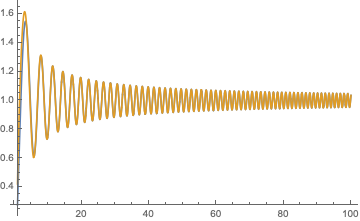# A numerical calculation for an integral

I am interested in the numerical calculation of $$F(\eta)=\frac{2}{π}\int_0^{+\infty}\sin(t\eta-t^3)\frac{dt}{t}\quad\text{for \eta\ge 0}.$$ I believe that the function $$F$$ is bounded, but I do not know its upper bound. It is easy to get $$F(0)=-1/3$$ and various other values for $$\eta \in [0,40]$$ but getting above the value $$40$$ for $$\eta$$ seems to raise computational difficulties for Mathematica. It is also interesting to see that $$F$$ takes values strictly above 1 with the seemingly largest value $$1.5$$ for $$\eta =3$$.

As a motivation, one may note that with $$g(t)= e^{it^3}\text{pv}\frac{1}{πt}$$, the operator of convolution with $$g$$ is bounded on $$L^2(\mathbb R)$$ whenever its Fourier transform is bounded. We have here $$F=\hat g$$, up to some normalization, and a precise bound in $$L^\infty$$ for $$F$$ would provide the $$\mathcal B(L^2)$$ operator-norm of that convolution.

• – user82588 Feb 10 at 17:12
• @Nemo Thank you very much for that precise piece of information. – Bazin Feb 11 at 10:05

Nemo's representation of $$F(\eta)$$ in terms of a hypergeometric function can be evaluated without difficulty for large $$\eta$$: $$F(\eta)=\frac{\sqrt{3} {\eta}^2 \Gamma \left(\frac{2}{3}\right) \; _1F_2\left(\frac{2}{3};\frac{4}{3},\frac{5}{3};-\frac{{\eta}^3}{27}\right)}{6\pi }-\frac{12 {\eta} \; _1F_2\left(\frac{1}{3};\frac{2}{3},\frac{4}{3};-\frac{{\eta}^3}{27}\right)}{6\Gamma \left(-\frac{1}{3}\right)}-\frac{1}{3}$$

$$F(\eta)\rightarrow 1+\sqrt{3} \sqrt{2/\pi}\frac{1}{\eta^{3/4}} \left[\sin \left(\frac{2 \eta^{3/2}}{3 \sqrt{3}}\right)-\cos \left(\frac{2 \eta^{3/2}}{3 \sqrt{3}}\right)\right],\;\;\eta\gg 1.$$

see plot, blue is $$F(\eta)$$, gold is the large-$$\eta$$ approximation (nearly indistinguishable for $$\eta>5$$). So $$F(\eta)$$ oscillates around 1, with an amplitude that decays as $$\eta^{-3/4}$$. The maximum 1.5487 is reached at $$\eta= 3.37213$$.• Great! Thank you very much. – Bazin Feb 11 at 9:14
• In your asymptotic expression, it seems that the term $\sqrt{2π}$ should be replaced by $\sqrt{2/π}$. – Bazin Feb 11 at 12:40
• Sorry to bother you again with that matter. I would be very grateful if you could double-check your constant $\sqrt{2\pi}$, which I believe should be replaced by $\sqrt{2/\pi}$. Thanks. – Bazin Feb 13 at 10:31
• you are right, it was my typing error, apologies for the confusion. – Carlo Beenakker Feb 13 at 10:42
• Thanks a lot for caring to check this; of course I renew my thanks for your very helpful and thorough answer to my question. – Bazin Feb 13 at 16:05